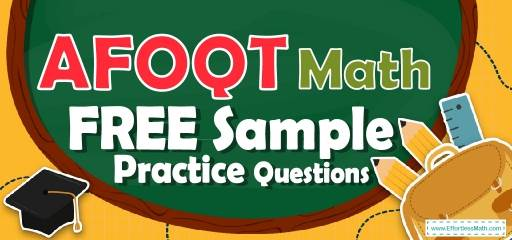# AFOQT Math FREE Sample Practice QuestionsPreparing for the AFOQT Math test? To succeed on the AFOQT Math test, you need to practice as many real AFOQT Math questions as possible.  There’s nothing like working on AFOQT Math sample questions to measure your exam readiness and put you more at ease when taking the AFOQT Math test. The sample math questions you’ll find here are brief samples designed to give you the insights you need to be as prepared as possible for your AFOQT Math test.

Check out our sample AFOQT Math practice questions to find out what areas you need to practice more before taking the AFOQT Math test!

Start preparing for the 2022 AFOQT Math test with our free sample practice questions. Also, make sure to follow some of the related links at the bottom of this post to get a better idea of what kind of mathematics questions you need to practice.

## 10 Sample AFOQT Math Practice Questions

1- A card is drawn at random from a standard $$52$$–card deck, what is the probability that the card is of Hearts? (The deck includes $$13$$ of each suit clubs, diamonds, hearts, and spades)

A. $$\frac{1}{3}$$

B. $$\frac{1}{6}$$

C. $$\frac{1}{52}$$

D. $$\frac{1}{4}$$

2- $$(5x + 5) (2x + 6) =$$ ?

A. $$5x + 6$$

B. $$10x^2 + 40x + 30$$

C. $$5x + 5x + 30$$

D. $$5x^2 + 5$$

3- The mean of $$50$$ test scores was calculated as $$88$$. But, it turned out that one of the scores was misread as $$94$$ but it was $$69$$. What is the mean?

A. $$85$$

B. $$87$$

C. $$87.5$$

D. $$88.5$$

4- $$5 (a – 6) = 22$$, what is the value of $$a$$?

A. $$2.4$$

B. $$10.4$$

C. $$7$$

D. $$11$$

5- If $$3^{24}=3^8× 3^x$$, what is the value of $$x$$?

A. $$2$$

B. $$1.5$$

C. $$3$$

D. $$16$$

6- Jason is $$9$$ miles ahead of Joe running at $$5.5$$ miles per hour and Joe is running at the speed of $$7$$ miles per hour. How long does it take Joe to catch Jason?

A. $$3$$ hours

B. $$4$$ hours

C. $$6$$ hours

D. $$8$$ hours

7- $$55$$ students took an exam and $$11$$ of them failed. What percent of the students passed the exam?

A. $$40\%$$

B. $$60\%$$

C. $$80\%$$

D. $$20\%$$

8- Factor this expression: $$x^2 + 5 − 6$$

A. $$x^2(5 + 6)$$

B. $$x(x + 5 – 6)$$

C. $$(x + 6)(x – 1)$$

D. $$(x + 6)(x – 6)$$

9- Find the slope of the line running through the points $$(6, 7)$$ and $$(5, 3)$$.

A. $$\frac{1}{4}$$

B. $$4$$

C. $$-4$$

D. $$-\frac{1}{4}$$

10- What is the missing term in the given sequence?
$$2$$, $$3$$, $$5$$, $$8$$, $$12$$, $$17$$, $$23$$, _, $$38$$

A. $$30$$

B. $$28$$

C. $$27$$

D. $$25$$

## Best AFOQT Math Prep Resource for 2022

1- D
The probability of choosing a Hearts is $$\frac{13}{52}=\frac{1}{4}$$

2- B
Use FOIL (first, out, in, last) method.
$$(5x + 5) (2x + 6) = 10x^2 + 30x + 10x + 30 = 10x^2 + 40x + 30$$

3- C
average (mean) $$=\frac{(sum \space of \space terms)}{(number \space of \space terms)} {\Rightarrow} 88 = \frac{(sum \space of \space terms)}{50} {\Rightarrow}$$sum $$= 88 {\times} 50 = 4400$$
The difference of $$94$$ and $$69$$ is $$25$$. Therefore, $$25$$ should be subtracted from the sum.
$$4400 – 25 = 4375$$
mean $$= \frac{(sum of terms)}{(number of terms)} ⇒$$ mean $$= \frac{(4375)}{50}= 87.5$$

4- B
$$5(a–6)=22 ⇒ 5a-30=22 ⇒5a=22+30=52$$
$$⇒ 5a=52⇒a=\frac{52}{5}= 10.4$$

5- D
Use exponent multiplication rule:
$$x^a . x^b = x^{a + b}$$
Then:
$$3^{24}=3^8× 3^x=3^{8+x}$$
$$24 = 8 + x ⇒x=24-8=16$$

6- C
The distance between Jason and Joe is $$9$$ miles. Jason running at $$5.5$$ miles per hour and Joe is running at the speed of $$7$$ miles per hour. Therefore, every hour the distance is $$1.5$$ miles less. $$9 \div 1.5 = 6$$

7- C
The failing rate is 11 out of $$55 = \frac{11}{55}$$
Change the fraction to percent:
$$\frac{11}{55} {\times} 100\%=20\%$$
$$20$$ percent of students failed. Therefore, $$80$$ percent of students passed the exam.

8- C
To factor the expression $$x^2 + 5 – 6$$, we need to find two numbers whose sum is $$5$$ and their product is $$-6$$.
Those numbers are $$6$$ and $$-1$$. Then:
$$x^2+5-6=(x+6)(x-1)$$

9- B
Slope of a line:$$\frac{y_2- y_1}{x_2 – x_1}=\frac{rise}{run}$$
$$\frac{y_2- y_1}{x_2 – x_1}=\frac{3- 7}{5 – 6}=\frac{-4}{-1}= 4$$

10- A
The difference of $$2$$ and $$3$$ is $$1$$, $$3$$ and $$5$$ is $$2$$, $$5$$ and $$8$$ is $$3$$, $$8$$ and $$12$$ is $$4$$, $$12$$ and $$17$$ is $$5$$, $$17$$ and $$23$$ is $$6$$, $$23$$ and next number should be $$7$$. The number is $$23 + 7 = 30$$

## More from Effortless Math for AFOQT Test …

### Need help preparing for the AFOQT Math test?

Check out our The Ultimate AFOQT Math Course.

### Looking for AFOQT Math FREE online resources?

Here is our complete list of Top 10 Websites for FREE AFOQT Math Preparation.

## Have any questions about the AFOQT Test?

### What people say about "AFOQT Math FREE Sample Practice Questions - Effortless Math: We Help Students Learn to LOVE Mathematics"?

No one replied yet.

X
52% OFF

Limited time only!

Save Over 52%

SAVE $40 It was$76.99 now it is \$36.99